# TSA Stationarity Test VI

LabVIEW 2014 Advanced Signal Processing Toolkit Help

Edition Date: June 2014

Part Number: 372656C-01

»View Product InfoDownload Help (Windows Only)

Owning Palette: Statistical Analysis VIs

Requires: Advanced Signal Processing Toolkit

Estimates the stationarity of a univariate time series by examining the mean and variance values of the subsequences. Wire data to the Xt input to determine the polymorphic instance to use or manually select the instance.

Use the pull-down menu to select an instance of this VI.

 Select an instance TSA Stationarity Test (Waveform)TSA Stationarity Test (Array)

## TSA Stationarity Test (Waveform)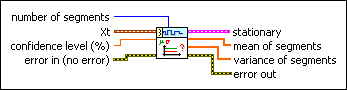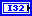number of segments specifies the number of subsequences into which this VI divides the input time series. The default is 100.Xt specifies the univariate time series.confidence level specifies the level of confidence as a percentage this VI uses to compute the confidence limits of specified statistics value.error in describes error conditions that occur before this node runs. This input provides standard error in functionality.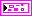stationary returns information about the stationarity of the univariate time series.mean? returns TRUE if the univariate time series is stationary based on the mean values of the subsequences.variance? returns TRUE if the univariate time series is stationary based on the variance values of the subsequences.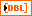mean of segments returns the mean value of each subsequence.variance of segments returns the variance value of each subsequence.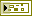error out contains error information. This output provides standard error out functionality.

## TSA Stationarity Test (Array)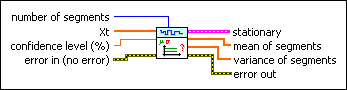number of segments specifies the number of subsequences into which this VI divides the input time series. The default is 100.Xt specifies the univariate time series.confidence level specifies the level of confidence as a percentage this VI uses to compute the confidence limits of specified statistics value.error in describes error conditions that occur before this node runs. This input provides standard error in functionality.stationary returns information about the stationarity of the univariate time series.mean? returns TRUE if the univariate time series is stationary based on the mean values of the subsequences.variance? returns TRUE if the univariate time series is stationary based on the variance values of the subsequences.mean of segments returns the mean value of each subsequence.variance of segments returns the variance value of each subsequence.error out contains error information. This output provides standard error out functionality.

## TSA Stationarity Test Details

This VI performs stationarity estimation on a univariate time series by testing the inversion number according to the following steps:

1. Divides a time series Xt into l subsequences. The mean value of each subsequence forms a time series m1, m2, …ml. The standard deviation value of each subsequence forms a time series s1, s2, …sl.

2. Computes the sum Sm (Ss) of inversion number for the time series m1, m2, …, ml (s1, s2, …, sl).

If Xt is stationary, the statistical value emes satisfies the normal distribution with a mean value of 0 and a standard deviation value of 1.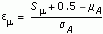andWhere mA is the theoretical mean value of Sm or Ss, which equals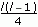, and sA is the theoretical standard deviation value of Sm or Ss, which equals the following equation: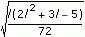Given the confidence level a:

• If em<Na/2(0, 1), this time series is mean stationary.
• If es<Na/2(0, 1), this time series is variance stationary.

### Reference:

Bendat, J.S., and A.G. Piersol. 2010. Random data analysis and measurement procedures. 4th edition. John Wiley and Sons.

## Example

Refer to the Series Statistical Analysis VI in the labview\examples\Time Series Analysis\TSAGettingStarted directory for an example of using the TSA Stationarity Test VI.

Not Helpful